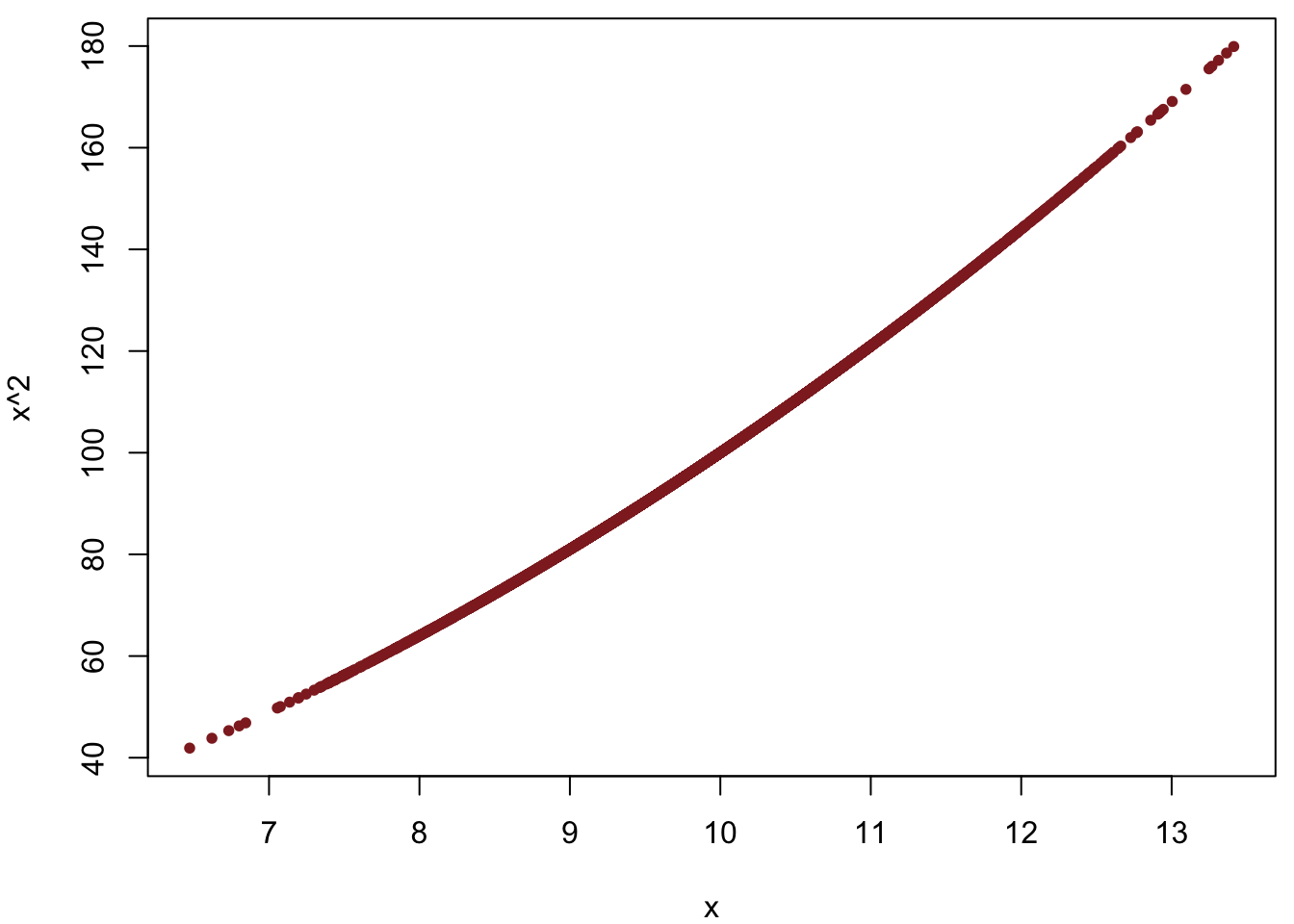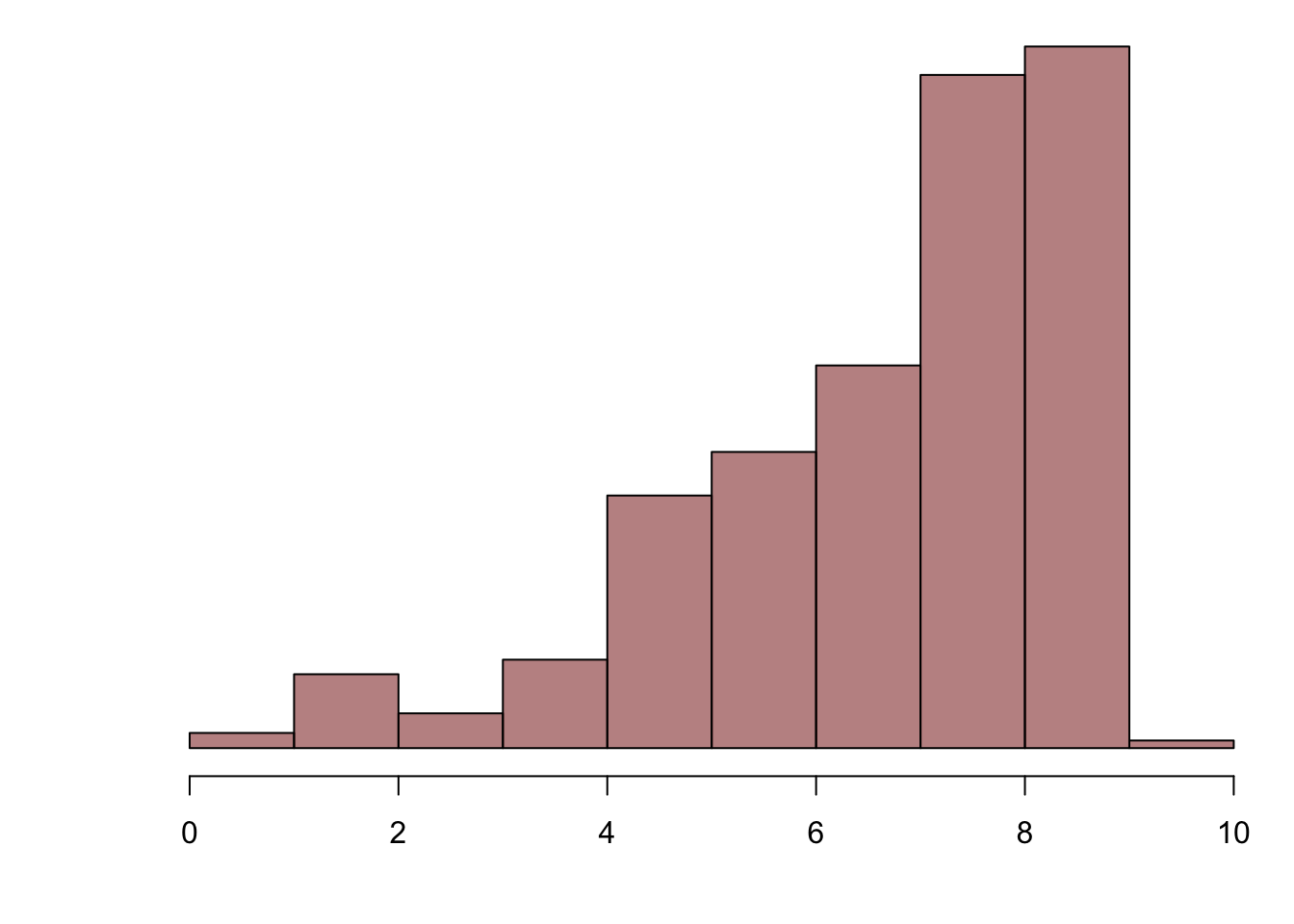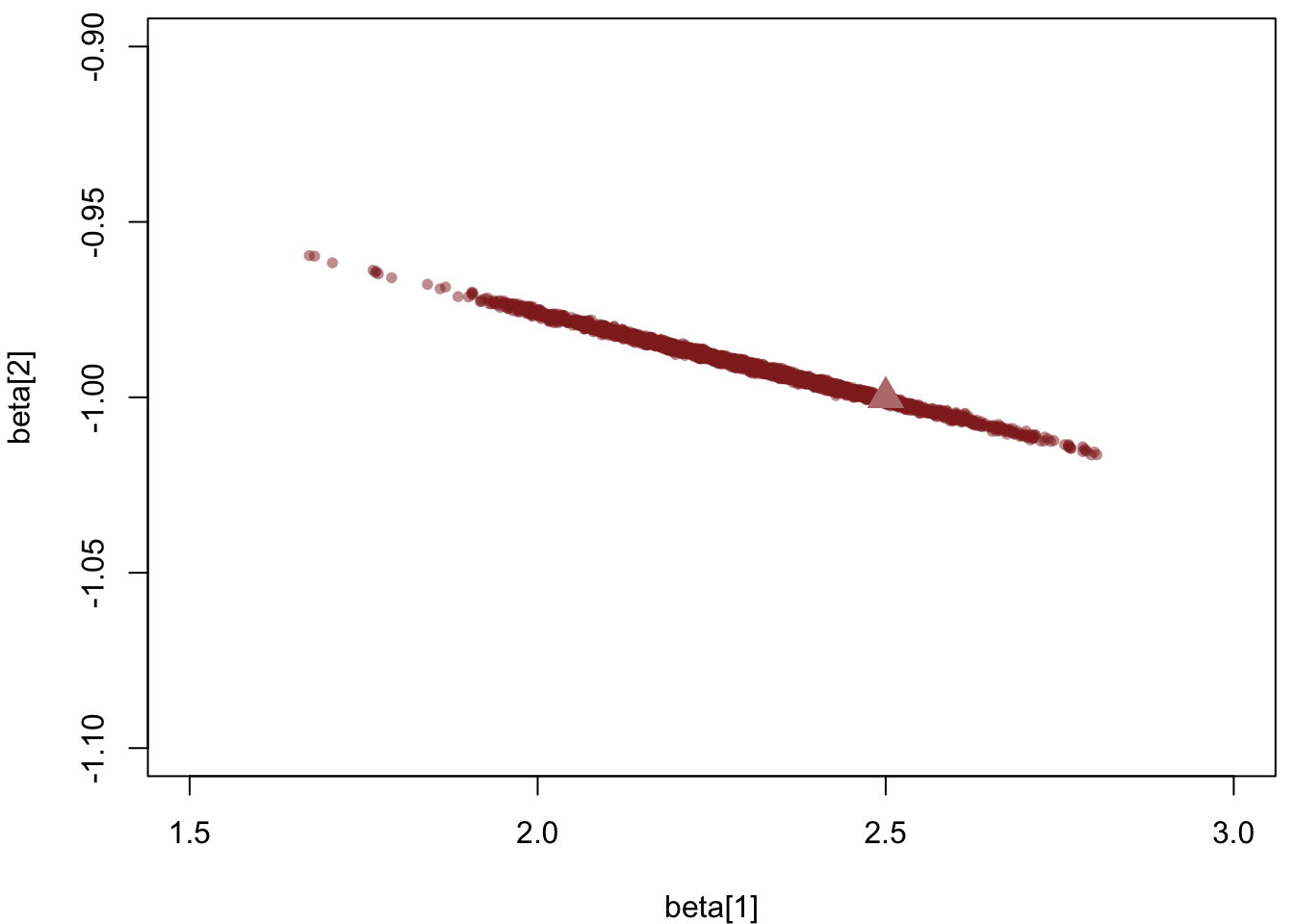A common problem in regression modeling is correlation amongst the covariates which can induce strong posterior correlations that frustrate accurate computation. In this case study I will review the QR decomposition, a technique for decorrelating covariates and, consequently, the resulting posterior distribution.

We’ll begin with a simple example that demonstrates the difficulties induced by correlated covariates before going through the mathematics of the QR decomposition and finally how it can be applied in Stan.

# Setting up the RStan Environment

First things first, let’s setup our local environment,

library(rstan)
Loading required package: ggplot2
Loading required package: StanHeaders
rstan (Version 2.14.1, packaged: 2016-12-28 14:55:41 UTC, GitRev: 5fa1e80eb817)
For execution on a local, multicore CPU with excess RAM we recommend calling
rstan_options(auto_write = TRUE)
options(mc.cores = parallel::detectCores())
rstan_options(auto_write = TRUE)
options(mc.cores = parallel::detectCores())
source("stan_utility.R")

c_light <- c("#DCBCBC")
c_light_highlight <- c("#C79999")
c_mid <- c("#B97C7C")
c_mid_highlight <- c("#A25050")
c_dark <- c("#8F2727")
c_dark_highlight <- c("#7C0000")

c_light_trans <- c("#DCBCBC80")
c_light_highlight_trans <- c("#C7999980")
c_mid_trans <- c("#B97C7C80")
c_mid_highlight_trans <- c("#A2505080")
c_dark_trans <- c("#8F272780")
c_dark_highlight_trans <- c("#7C000080")

# Fitting Issues with Correlated Covariates

Now consider a very simple regression with only two covariates – $$x \sim \mathcal{N} (1, 0.1)$$ and it’s square, $$x^{2}$$. The inclusion of both $$x$$ and $$x^{2}$$ is not uncommon in polynomial regressions where the response is given by a sum of polynomials over the input covariates.

In particular, the correlations here are particularly strong because we didn’t standardize the covariate, $$x$$, before squaring it. Powers are much better identified when the input is centered around zero. Unfortunately in practice we may not be able to standardize the covariates before they are transformed. Moreover, in more complex regressions seemingly independent covariates are often highly correlated due to common confounders in which case standardization will not be of much help.

We begin by simulating some data and, per good Stan practice, saving it in an external file,

set.seed(689934)

N <- 5000
x <- rnorm(N, 10, 1)
X = t(data.matrix(data.frame(x, x * x)))

M <- 2
beta = matrix(c(2.5, -1), nrow=M, ncol=1)
alpha <- -0.275
sigma <- 0.8

mu <- t(X) %*% beta + alpha
y = sapply(1:N, function(n) rnorm(1, mu[n], sigma))

stan_rdump(c("N", "M", "X", "y"), file="regr.data.R")

Because the covariate $$x$$ is restricted to positive values, it is highly correlated with its square,

par(mar = c(4, 4, 0.5, 0.5))
plot(X[1,], X[2,],
col=c_dark, pch=16, cex=0.8, xlab="x", ylab="x^2")With the data in hand we can attempt to fit a naive linear regression model,

writeLines(readLines("regr.stan"))
data {
int<lower=1> N;
int<lower=1> M;
matrix[M, N] X;
vector[N] y;
}

parameters {
vector[M] beta;
real alpha;
real<lower=0> sigma;
}

model {
beta ~ normal(0, 10);
alpha ~ normal(0, 10);
sigma ~ cauchy(0, 10);

y ~ normal(X' * beta + alpha, sigma);
}
input_data <- read_rdump("regr.data.R")
fit <- stan(file='regr.stan', data=input_data, seed=483892929)

Checking our diagnostics,

print(fit)
Inference for Stan model: regr.
4 chains, each with iter=2000; warmup=1000; thin=1;
post-warmup draws per chain=1000, total post-warmup draws=4000.

mean se_mean   sd     2.5%      25%      50%      75%    97.5%
beta     2.31    0.01 0.16     2.00     2.20     2.30     2.42     2.63
beta    -0.99    0.00 0.01    -1.01    -1.00    -0.99    -0.99    -0.98
alpha       0.76    0.03 0.80    -0.82     0.22     0.79     1.31     2.33
sigma       0.81    0.00 0.01     0.79     0.80     0.81     0.81     0.82
lp__    -1432.46    0.04 1.38 -1435.82 -1433.15 -1432.15 -1431.44 -1430.71
n_eff Rhat
beta   715 1.00
beta   719 1.00
alpha     718 1.00
sigma    1532 1.00
lp__     1209 1.01

Samples were drawn using NUTS(diag_e) at Thu Jul 27 22:52:22 2017.
For each parameter, n_eff is a crude measure of effective sample size,
and Rhat is the potential scale reduction factor on split chains (at
convergence, Rhat=1).
check_treedepth(fit)
 "12 of 4000 iterations saturated the maximum tree depth of 10 (0.3%)"
 "Run again with max_depth set to a larger value to avoid saturation"
check_energy(fit)
check_div(fit)
 "0 of 4000 iterations ended with a divergence (0%)"

we see that everything looks okay save for some trajectories that have prematurely terminated because of the default tree depth limit. Although there aren’t many of them,

breaks <- 0:10
sampler_params <- get_sampler_params(fit, inc_warmup=FALSE)
treedepths <- do.call(rbind, sampler_params)[,'treedepth__']
treedepths_hist <- hist(treedepths, breaks=breaks, plot=FALSE)

par(mar = c(4, 4, 0.5, 0.5))
plot(treedepths_hist, col=c_dark_highlight_trans, main="",
xlab="theta.1", yaxt='n', ann=FALSE)even a few prematurely terminating trajectories can hinder performance, and may even indicate potential problems with adaptation.

To maximize performance and avoid any potential issues we refit with a larger tree depth threshold,

fit <- stan(file='regr.stan', data=input_data, seed=483892929, control=list(max_treedepth=15))

Now the diagnostics are clean

print(fit)
Inference for Stan model: regr.
4 chains, each with iter=2000; warmup=1000; thin=1;
post-warmup draws per chain=1000, total post-warmup draws=4000.

mean se_mean   sd     2.5%      25%      50%      75%    97.5%
beta     2.31    0.01 0.16     2.00     2.20     2.30     2.42     2.63
beta    -0.99    0.00 0.01    -1.01    -1.00    -0.99    -0.99    -0.98
alpha       0.76    0.03 0.80    -0.82     0.22     0.79     1.31     2.33
sigma       0.81    0.00 0.01     0.79     0.80     0.81     0.81     0.82
lp__    -1432.46    0.04 1.38 -1435.82 -1433.15 -1432.15 -1431.44 -1430.71
n_eff Rhat
beta   715 1.00
beta   719 1.00
alpha     718 1.00
sigma    1532 1.00
lp__     1209 1.01

Samples were drawn using NUTS(diag_e) at Thu Jul 27 22:52:22 2017.
For each parameter, n_eff is a crude measure of effective sample size,
and Rhat is the potential scale reduction factor on split chains (at
convergence, Rhat=1).
check_treedepth(fit, 15)
 "0 of 4000 iterations saturated the maximum tree depth of 15 (0%)"
check_energy(fit)
check_div(fit)
 "0 of 4000 iterations ended with a divergence (0%)"

The small step sizes, however,

sampler_params <- get_sampler_params(fit, inc_warmup=FALSE)
stepsizes <- sapply(sampler_params, function(x) x[1,'stepsize__'])
names(stepsizes) <- list("Chain 1", "Chain 2", "Chain 3" ,"Chain 4")
stepsizes
    Chain 1     Chain 2     Chain 3     Chain 4
0.007370508 0.006502418 0.006522210 0.007188796 

do require significant computation, over one million gradient evaluations, across the entire fit,

n_gradients <- sapply(sampler_params, function(x) sum(x[,'n_leapfrog__']))
n_gradients
 332728 329700 334262 321326
sum(n_gradients)
 1318016

Plotting the posterior samples we can see why. The marginal posterior for the slopes is only weakly-identified and the posterior geometry becomes extremely narrow,

partition <- partition_div(fit)
params <- partition[]

par(mar = c(4, 4, 0.5, 0.5))
plot(params$'beta', params$'beta',
col=c_dark_trans, pch=16, cex=0.8, xlab="beta", ylab="beta",
xlim=c(1.5, 3), ylim=c(-1.1, -0.9))
points(beta[1,1], beta[2,1],
col=c_mid, pch=17, cex=2)requiring very precise trajectory simulations at each iteration of the Markov chains.

# Decorrelating the Posterior with a QR Decomposition

Fortunately we can reduce the correlations between the covariates, and ameliorate the challenging geometry of the Bayesian posterior, by applying a QR decomposition. Perhaps unsurprisingly this is the same QR decomposition that arises in the analytic maximum likelihood and conjugate Bayesian treatment of linear regression, although here it will be applicable regardless of the choice of priors and for any general linear model.

## Mathematical Derivation

The thin QR decomposition decomposes a rectangular $$N \times M$$ matrix into $\mathbf{A} = \mathbf{Q} \cdot \mathbf{R}$ where $$\mathbf{Q}$$ is an $$N \times M$$ orthogonal matrix with $$M$$ non-zero rows and $$N - M$$ rows of vanishing rows, and $$\mathbf{R}$$ is a $$M \times M$$ upper-triangular matrix.

If we apply the decomposition to the transposed design matrix, $$\mathbf{X}^{T} = \mathbf{Q} \cdot \mathbf{R}$$, then we can refactor the linear response as \begin{align*} \boldsymbol{\mu} &= \mathbf{X}^{T} \cdot \boldsymbol{\beta} + \alpha \\ &= \mathbf{Q} \cdot \mathbf{R} \cdot \boldsymbol{\beta} + \alpha \\ &= \mathbf{Q} \cdot (\mathbf{R} \cdot \boldsymbol{\beta}) + \alpha \\ &= \mathbf{Q} \cdot \widetilde{\boldsymbol{\beta}} + \alpha. \\ \end{align*}

Because the matrix $$\mathbf{Q}$$ is orthogonal, its columns are independent and consequently we expect the posterior over the new parameters, $$\widetilde{\boldsymbol{\beta}} = \mathbf{R} \cdot \boldsymbol{\beta}$$, to be significantly less correlated. In practice we can also equalize the scales of the posterior by normalizing the $$Q$$ and $$R$$ matrices, \begin{align*} \mathbf{Q} &\rightarrow \mathbf{Q} \cdot N \\ \mathbf{R} &\rightarrow \mathbf{R} \, / \, N. \end{align*}

We can then readily recover the original slopes as $\boldsymbol{\beta} = \mathbf{R}^{-1} \cdot \widetilde{\boldsymbol{\beta}}.$ As $$\mathbf{R}$$ is upper diagonal we could compute its inverse with only $$\mathcal{O} (M^{2})$$ operations, but because we need to compute it only once we will use the naive inversion function in Stan here.

Because the transformation between $$\boldsymbol{\beta}$$ and $$\widetilde{\boldsymbol{\beta}}$$ is linear, the corresponding Jacobian depends only on the data and hence doesn’t affect posterior computations. This means that in Stan we can define the transformed parameters $$\boldsymbol{\beta} = \mathbf{R}^{-1} \cdot \widetilde{\boldsymbol{\beta}}$$ and apply priors directly to $$\boldsymbol{\beta}$$ while ignoring the warning about Jacobians.

Interestingly, applying weakly-informative priors to the $$\widetilde{\boldsymbol{\beta}}$$ directly can be interpreted as a form of empirical Bayes, where we use the empirical correlations in the data to guide the choice of prior.

## Implementation in Stan

The scaled, thin QR decomposition is straightforward to implement in Stan,

writeLines(readLines("qr_regr.stan"))
data {
int<lower=1> N;
int<lower=1> M;
matrix[M, N] X;
vector[N] y;
}

transformed data {
// Compute, thin, and then scale QR decomposition
matrix[N, M] Q = qr_Q(X')[, 1:M] * N;
matrix[M, M] R = qr_R(X')[1:M, ] / N;
matrix[M, M] R_inv = inverse(R);
}

parameters {
vector[M] beta_tilde;
real alpha;
real<lower=0> sigma;
}

transformed parameters {
vector[M] beta = R_inv * beta_tilde;
}

model {
beta ~ normal(0, 10);
alpha ~ normal(0, 10);
sigma ~ cauchy(0, 10);

y ~ normal(Q * beta_tilde + alpha, sigma);
}

Fitting the QR regression model, and ignoring the warning about the Jacobian due to the considerations above,

qr_fit <- stan(file='qr_regr.stan', data=input_data, seed=483892929)

we see no indications of an inaccurate fit,

print(qr_fit)
Inference for Stan model: qr_regr.
4 chains, each with iter=2000; warmup=1000; thin=1;
post-warmup draws per chain=1000, total post-warmup draws=4000.

mean se_mean   sd     2.5%      25%      50%      75%
beta_tilde    -1.11    0.00 0.01    -1.13    -1.12    -1.11    -1.10
beta_tilde    -0.14    0.00 0.00    -0.14    -0.14    -0.14    -0.14
alpha             0.76    0.03 0.88    -0.93     0.14     0.76     1.36
sigma             0.81    0.00 0.01     0.79     0.80     0.81     0.81
beta           2.31    0.01 0.18     1.96     2.19     2.31     2.44
beta          -0.99    0.00 0.01    -1.01    -1.00    -0.99    -0.99
lp__          -1432.58    0.05 1.47 -1436.35 -1433.32 -1432.25 -1431.50
97.5% n_eff Rhat
beta_tilde    -1.09   716    1
beta_tilde    -0.14   724    1
alpha             2.51   715    1
sigma             0.82  1266    1
beta           2.65   718    1
beta          -0.97   724    1
lp__          -1430.75   946    1

Samples were drawn using NUTS(diag_e) at Thu Jul 27 22:54:26 2017.
For each parameter, n_eff is a crude measure of effective sample size,
and Rhat is the potential scale reduction factor on split chains (at
convergence, Rhat=1).
check_treedepth(qr_fit)
 "0 of 4000 iterations saturated the maximum tree depth of 10 (0%)"
check_energy(qr_fit)
check_div(qr_fit)
 "0 of 4000 iterations ended with a divergence (0%)"

The effective sample sizes are the same, but the larger step sizes,

sampler_params <- get_sampler_params(qr_fit, inc_warmup=FALSE)
qr_stepsizes <- sapply(sampler_params, function(x) x[1,'stepsize__'])
names(qr_stepsizes) <- list("Chain 1", "Chain 2", "Chain 3" ,"Chain 4")
qr_stepsizes
    Chain 1     Chain 2     Chain 3     Chain 4
0.009789558 0.012880644 0.011090654 0.011874532 

require only about half the gradient evaluations needed in the naive regression,

n_gradients <- sapply(sampler_params, function(x) sum(x[,'n_leapfrog__']))
n_gradients
 200248 169188 183426 170156
sum(n_gradients)
 723018

Consequently even in this simple example the QR decomposition is about twice as fast as the naive regression. In more complex, higher-dimensional regressions the improvement can be even larger.

This is not unexpected, however, given how much less correlated the posterior for the transformed slopes is,

partition <- partition_div(qr_fit)
params <- partition[]

par(mar = c(4, 4, 0.5, 0.5))
plot(params$'beta_tilde', params$'beta_tilde',
col=c_dark_trans, pch=16, cex=0.8, xlab="beta_tilde", ylab="beta_tilde")